6. 队列（Queue）# 6. 队列（Queue）

## 6.0. 什么是队列？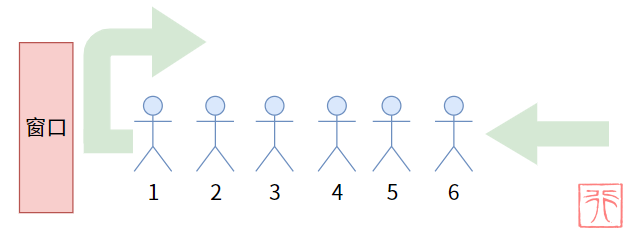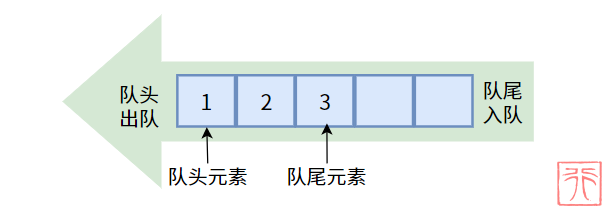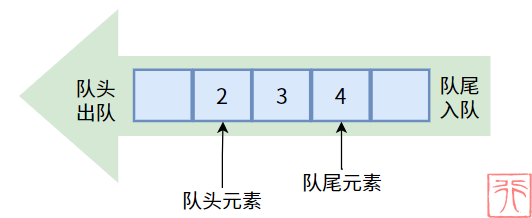• 入队：进入队列，即向队列中插入元素
• 出队：离开队列，即从队列中删除元素
• 队头：允许出队（删除）的一端
• 队尾：允许入队（插入）的一端
• 队头元素：队列中最先入栈的元素
• 队尾元素：队列中最后入栈的元素

## 6.1. 队列的实现思路

### 6.1.0. 数组实现——顺序队列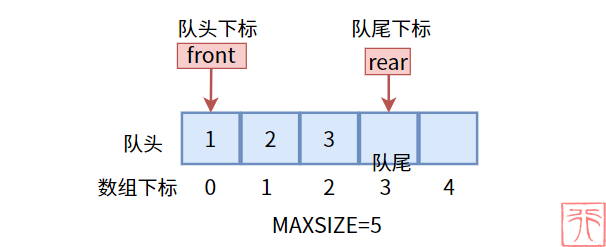• 存储数据的数组 —— `data[]`
• 表示队列的最大容量的值 —— `MAXSIZE`
• 标识队头端的队头下标 —— `front`
• 标识队尾端的队尾下标 —— `rear`

`front``rear` 会随着入队和出队操作而变化，为了方便起见，我们规定在非空队列中，队尾下标是队尾元素的下一个元素的下标

``````#define MAXSIZE 5 //顺序队列的最大存储容量
/*顺序队列的结构体*/
typedef struct {
int data[MAXSIZE];
int front; //队头下标
int rear; //队尾下标
} QueueArray;``````

### 6.1.1. 链表实现——链队列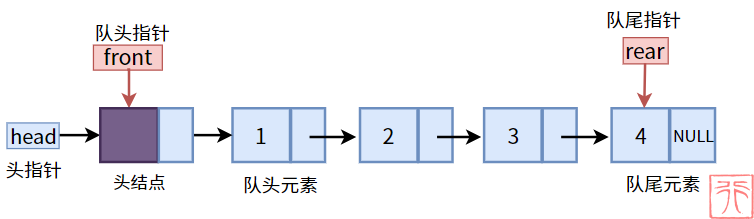• 单链表的基本单元结点 —— `QueueNode`
• 存储数据的数据域 —— `data`
• 指向下一个结点的指针域 —— `next`
• 指向链表的头指针 —— `head`
• 标识队头端的队头指针 —— `front`
• 标识队尾端的队尾指针 —— `rear`

``````/*单链表的结点的结构体*/
typedef struct QueueNode {
int data; //数据域
struct QueueNode *next; //指针域
} QueueNode;

/*链队列的结构体*/
typedef struct {
QueueNode *front; //队头指针
QueueNode *rear; //队尾指针

## 6.2. 队列的状态

### 6.2.0. 顺序队列（问题版）

【空队列】：空队列中没有元素，此时，队头下标和队尾下标均为 0，即`front = rear = 0`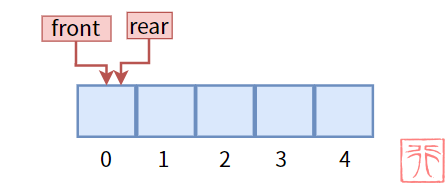【非空非满队列】：队列不是空队列且有剩余空间：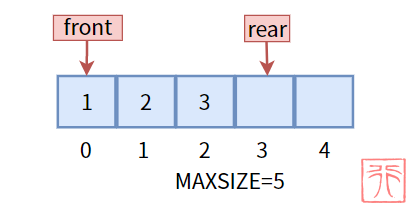【满队列】：顺序队列分配的固定空间用尽，没有多余空间，不能再插入元素，此时 `front = 0``rear = MAXSIZE`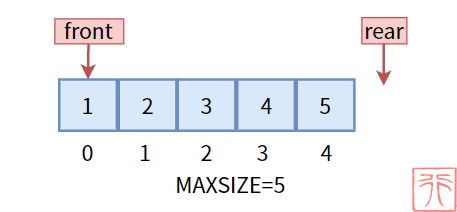### 6.2.1. 假满队列

• 队列只允许在队头删除元素，在队尾插入元素
• 我们规定：`front` 是队头元素的下标，`rear` 是队尾元素的下标，二者会随着出队和入队操作而变化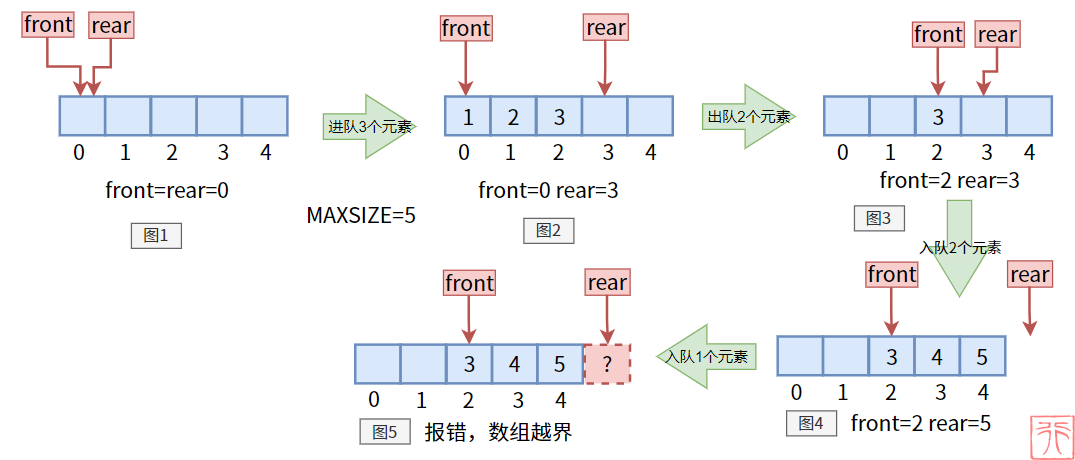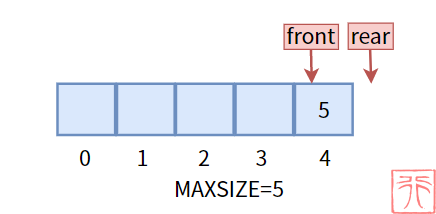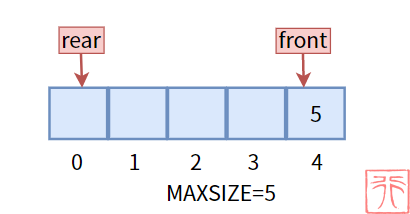### 6.2.2. 循环队列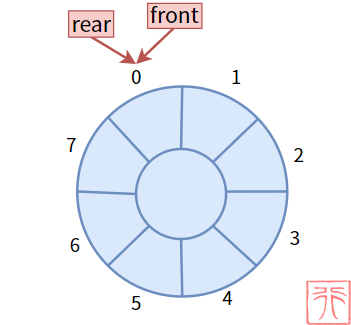【空队列】：队列中没有元素，如上图。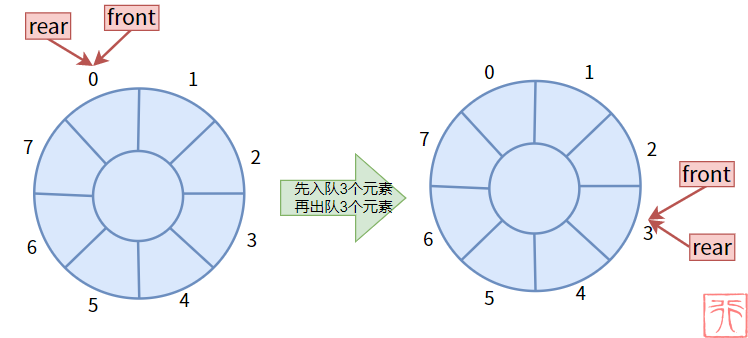【满队列】：队列中没有空闲空间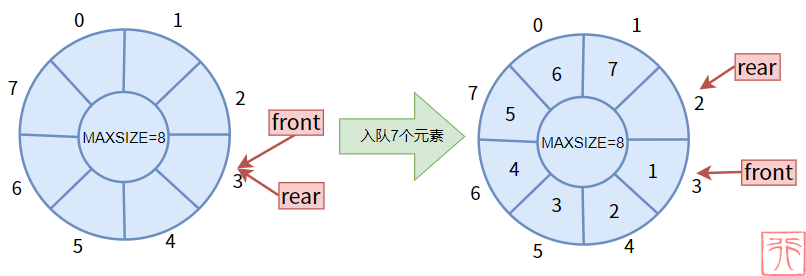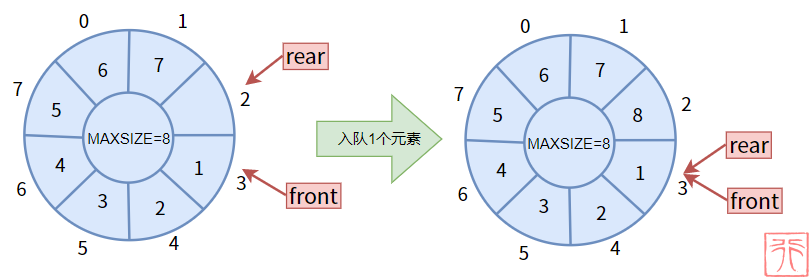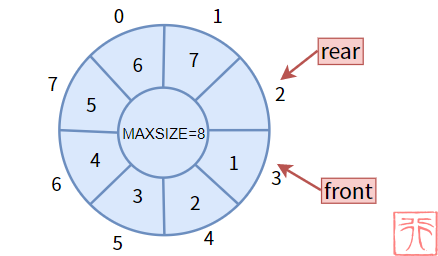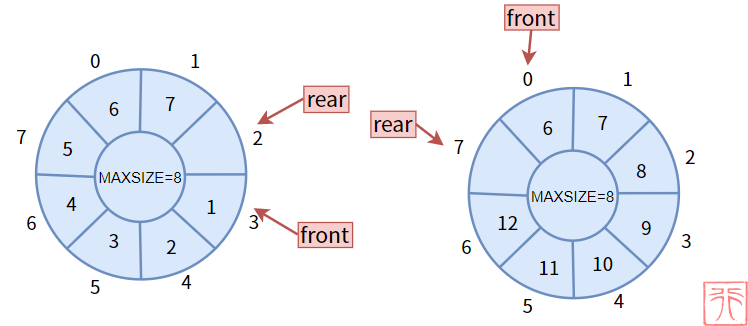【非空非满队列】 很好理解，不再赘述。

### 6.2.3. 链队列

【空队列】：即一个空链表，此时队头指针（兼链表头指针）和队尾指针均指向头结点。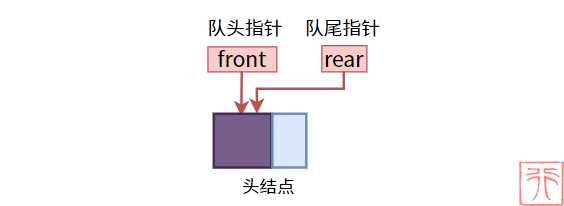【非空队列】：不像顺序队列那样有空间的限制，链队列的空间是不受限制的（只要你的内存足够大），所以自然不存在“满队列”“循环队列”的概念。

## 6.3. 初始化

### 6.3.0. 顺序队列

``````/**
* 初始化顺序队列：将队头下标和队尾下标置为0
* queue: 指向队列的指针
*/
void init(QueueArray *queue)
{
queue->front = 0;
queue->rear = 0;
}``````

### 6.3.1. 链队列

``````/**
* 初始化链队列：将队头指针和队尾指针指向头结点
*/
{
//创造头结点
//队头指针 队尾指针指向头结点
}``````

## 6.4. 入队操作

### 6.4.0. 顺序队列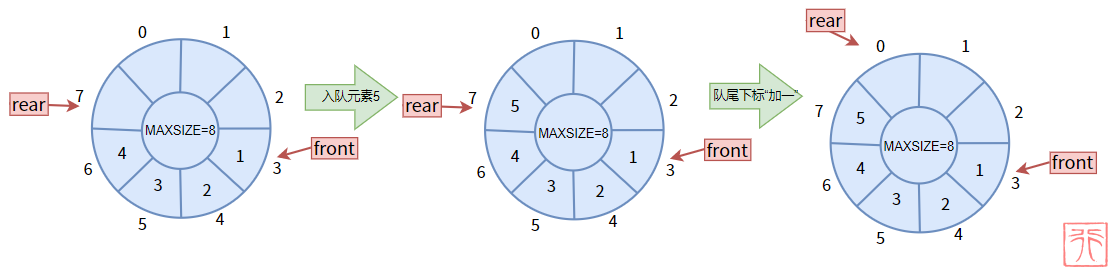``````/**
* 入队操作
* queue: 指向队列的指针
* elem: 入队的数据
* return: 0失败，1成功
*/
int en_queue(QueueArray *queue, int elem)
{
//判断队列是否已满
if ((queue->rear + 1) % MAXSIZE == queue->front) {
printf("队列已满，无法继续入队。\n");
return 0;
}
//元素入队
queue->data[queue->rear] = elem;
//队尾下标加一
queue->rear = (queue->rear + 1) % MAXSIZE;
return 1;
}``````

### 6.4.1. 链队列

``````/** * 入队操作
* queue: 指向队列的指针
* elem: 入队的数据
*/
{
//创造新结点
QueueNode *new = create_node(elem);
//入队（尾插法）
queue->rear->next = new;
queue->rear = new;
}``````

## 6.5. 出队操作

### 6.5.0. 顺序队列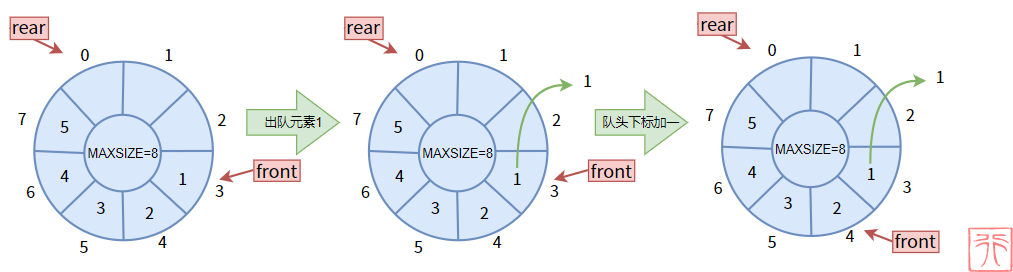``````/**
* 出队操作
* queue: 指向队列的指针
* elem: 指向保存出队数据的变量
* return: 0失败，1成功
*/
int de_queue(QueueArray *queue, int *elem)
{
//判读队列是否为空
if (queue->front == queue->rear) {
printf("队列空，无元素可出。\n");
return 0;
}
//元素出队
*elem = queue->data[queue->front];
//队头下标加一
queue->front = (queue->front + 1) % MAXSIZE;
return 1;
}``````

### 6.5.1. 链队列

``````/**
* 出队操作
* queue: 指向队列的指针
* elem: 指向保存变量的指针
* return: 0失败，1成功
*/
{
//判读队列是否为空
if (queue->front == queue->rear) {
printf("队列空，无元素可出。\n");
return 0;
}
QueueNode *front_node = queue->front->next; //队头元素
//保存数据
*elem = front_node->data;
//队头元素出队（头删法）
queue->front->next = front_node->next;
//如果元素出完，队尾指针重新指向头结点
if (front_node == queue->rear)
queue->rear = queue->front;
free(front_node);
}``````

## 6.6. 遍历操作

### 6.6.0. 顺序队列

``````/**
* 打印队列
*/
void output(QueueArray queue)
{
int i = queue.front;
while (i % MAXSIZE != queue.rear) {
printf("%d ", queue.data[i]);
i = (i + 1) % MAXSIZE;
}
printf("\n");
}``````• 下标 0 到 `rear`，长度为 `rear - 0`
• 下标 `MAXSIZE - 1``rear`，长度为 `MAXSIZE - front`

### 6.6.1. 链队列

``````/**
* 打印队列
*/
{
QueueNode *p = queue->front->next; //p指向队头元素
while (p != NULL) {
printf("%d ", p->data);
p = p->next;
}
printf("\n");
}``````22
Lv1
……
16

6

3